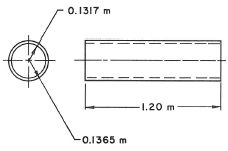Magnetodynamic TimeThe magnetodynamic solution describes the distribution of magnetic fields and eddy currents due to excitation frequencies.

We use a quasi stationary approximation. The assumptions are applicable in cases of dimensions << wavelength. Examples are motors, transformers and frequencies from 0 Hz to a few 100 kHz.

Features

Outputs of the solution are:
• Plot: Magnetic Fluxdensity, Magnetic Fieldstrength, Current Density, Eddy Current Losses Density, Vectorpotential, Nodal Force - virtual, Nodal Moment - virtual, Lorentz Force, Displacement, Error Estimation.
• Table: Total Force - virtual, Total Moment - virtual, Total Lorentz Force, RotorBand Torque - stresstensor, RotorBand Force - stresstensor, Vectorpotential on Conductors - Fluxlinkage, Voltage on Coils, Voltage on Circuits, Electrode Voltage, Electrode Current, Current on Circuits, Power on Circuits, Eddy Current Losses, Ohm Resistance, Coil Inductivity, Phase Shift.
• NVH Coupling: Forces in time and frequency domain on teeth.
• 4D Fields:  Force - virtual NodeID Table, Forcedensity - virtual XYZ Table, Lorentz Force NodeID Table.
• Coupled Thermal: Temperature

Theory and Basics

Formulations

The basis equations:
(1)        rot h = j
(2)        rot e = -δt b
(3)        div b = 0

Constitutive relations:
(4)        b = µ h
(5)        j = σ e

a-Formulation

The following a-formulation is used for 2D-Magnetodynamics.

Magnetic vectorpotential a:
(6)        b = rot a
(7)        e = -δt a

Magnetodynamic  weak  a-formulation:
(8)            ( µ-1 rot a, rot a’ )Ω
+ (-µ-1 bs, rot a’ ) Ω

+ (- ja’ ) ΩC
+ (σ δt aa’ ) Ωc

= 0, for all a’ element of Ω

a-v-Formulation

The following a-v-formulation is used for 3D-Magnetodynamics.

Magnetic vectorpotential a
electric scalar potential v:
(9)        b = rot a
(10)      e = -δt – grad v

Magnetodynamic  weak  a-v-formulation:
(11)            (µ-1 rot a, rot a’ )Ω
+ (σ δt aa’ ) Ωc
+ (σ grad v, a’ ) Ωc
+ (σ δt a, grad v’ ) Ωc

+ (σ grad v, v’ ) Ωc

= 0

Basic Example: Team Problem 1a: The Felix Cylinder

This example is a test case for electromagnetic analysis software tools. There are measured results available that will be used for comparison of our results.
The problem consists of a thin wall long hollow aluminum cylinder placed in a uniform magnetic field. The magnetic field is perpendicular to the axis of the cylinder and decays exponentially with time. The problem is to calculate the induced eddy currents at various axial positions.Mesh of a quarter of the geometry.Result

Eddy Currents over Time

The graph below shows the simulated Eddy Currents over the time.The reference result from the team benchmark. We have to compare the Z=0.5 m curve that is responsible for the section of the tube in the middle